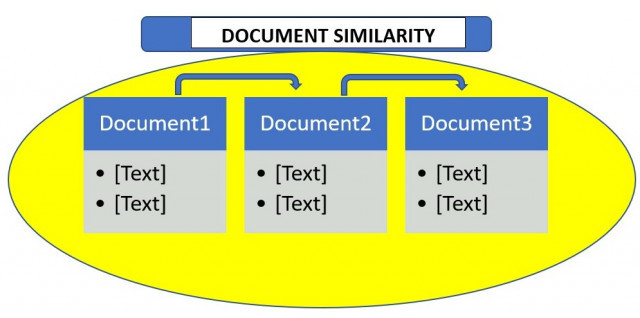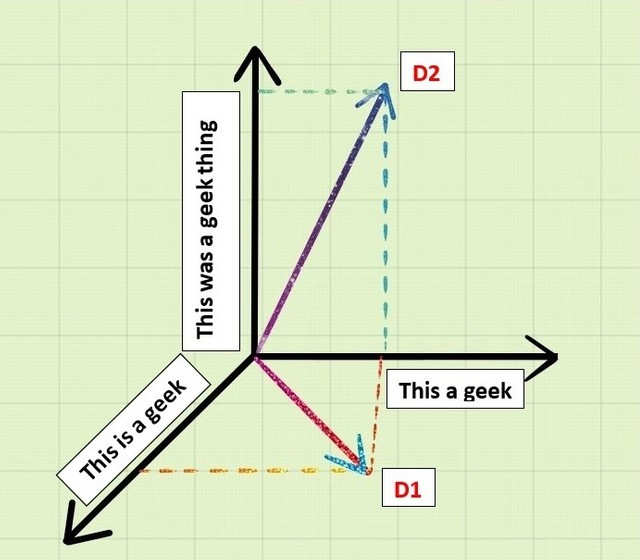Open in App
Not now

# Measuring the Document Similarity in Python

• Difficulty Level : Expert
• Last Updated : 27 Feb, 2020

Document similarity, as the name suggests determines how similar are the two given documents. By “documents”, we mean a collection of strings. For example, an essay or a .txt file. Many organizations use this principle of document similarity to check plagiarism. It is also used by many exams conducting institutions to check if a student cheated from the other. Therefore, it is very important as well as interesting to know how all of this works.Document similarity is calculated by calculating document distance. Document distance is a concept where words(documents) are treated as vectors and is calculated as the angle between two given document vectors. Document vectors are the frequency of occurrences of words in a given document. Let’s see an example:

Say that we are given two documents D1 and D2 as:

D1: “This is a geek”
D2: “This was a geek thing”

The similar words in both these documents then become:

`"This a geek"`

If we make a 3-D representation of this as vectors by taking D1, D2 and similar words in 3 axis geometry, then we get:Now if we take dot product of D1 and D2,

`D1.D2 = "This"."This"+"is"."was"+"a"."a"+"geek"."geek"+"thing".0`
`D1.D2 = 1+0+1+1+0`
`D1.D2 = 3`

Now that we know how to calculate the dot product of these documents, we can now calculate the angle between the document vectors:

`cos d = D1.D2/|D1||D2|`

Here d is the document distance. It’s value ranges from 0 degree to 90 degrees. Where 0 degree means the two documents are exactly identical and 90 degrees indicate that the two documents are very different.

Now that we know about document similarity and document distance, let’s look at a Python program to calculate the same:

Document similarity program :

Our algorithm to confirm document similarity will consist of three fundamental steps:

• Split the documents in words.
• Compute the word frequencies.
• Calculate the dot product of the document vectors.

For the first step, we will first use the `.read()` method to open and read the content of the files. As we read the contents, we will split them into a list. Next, we will calculate the word frequency list of the read in the file. Therefore, the occurrence of each word is counted and the list is sorted alphabetically.

 `import` `math ` `import` `string ` `import` `sys ` ` `  `# reading the text file ` `# This functio will return a  ` `# list of the lines of text  ` `# in the file. ` `def` `read_file(filename):  ` `     `  `    ``try``: ` `        ``with ``open``(filename, ``'r'``) as f: ` `            ``data ``=` `f.read() ` `        ``return` `data ` `     `  `    ``except` `IOError: ` `        ``print``(``"Error opening or reading input file: "``, filename) ` `        ``sys.exit() ` ` `  `# splitting the text lines into words ` `# translation table is a global variable ` `# mapping upper case to lower case and ` `# punctuation to spaces ` `translation_table ``=` `str``.maketrans(string.punctuation``+``string.ascii_uppercase, ` `                                     ``" "``*``len``(string.punctuation)``+``string.ascii_lowercase) ` `      `  `# returns a list of the words ` `# in the file ` `def` `get_words_from_line_list(text):  ` `     `  `    ``text ``=` `text.translate(translation_table) ` `    ``word_list ``=` `text.split() ` `     `  `    ``return` `word_list `

Now that we have the word list, we will now calculate the frequency of occurrences of the words.

 `# counts frequency of each word ` `# returns a dictionary which maps ` `# the words to  their frequency. ` `def` `count_frequency(word_list):  ` `     `  `    ``D ``=` `{} ` `     `  `    ``for` `new_word ``in` `word_list: ` `         `  `        ``if` `new_word ``in` `D: ` `            ``D[new_word] ``=` `D[new_word] ``+` `1` `             `  `        ``else``: ` `            ``D[new_word] ``=` `1` `             `  `    ``return` `D ` ` `  `# returns dictionary of (word, frequency) ` `# pairs from the previous dictionary. ` `def` `word_frequencies_for_file(filename):  ` `     `  `    ``line_list ``=` `read_file(filename) ` `    ``word_list ``=` `get_words_from_line_list(line_list) ` `    ``freq_mapping ``=` `count_frequency(word_list) ` ` `  `    ``print``(``"File"``, filename, ``":"``, ) ` `    ``print``(``len``(line_list), ``"lines, "``, ) ` `    ``print``(``len``(word_list), ``"words, "``, ) ` `    ``print``(``len``(freq_mapping), ``"distinct words"``) ` ` `  `    ``return` `freq_mapping `

Lastly, we will calculate the dot product to give the document distance.

 `# returns the dot product of two documents ` `def` `dotProduct(D1, D2):  ` `    ``Sum` `=` `0.0` `     `  `    ``for` `key ``in` `D1: ` `         `  `        ``if` `key ``in` `D2: ` `            ``Sum` `+``=` `(D1[key] ``*` `D2[key]) ` `             `  `    ``return` `Sum` ` `  `# returns the angle in radians  ` `# between document vectors ` `def` `vector_angle(D1, D2):  ` `    ``numerator ``=` `dotProduct(D1, D2) ` `    ``denominator ``=` `math.sqrt(dotProduct(D1, D1)``*``dotProduct(D2, D2)) ` `     `  `    ``return` `math.acos(numerator ``/` `denominator) `

That’s all! Time to see the document similarity function:

 `def` `documentSimilarity(filename_1, filename_2): ` `     `  `   ``# filename_1 = sys.argv ` `   ``# filename_2 = sys.argv ` `    ``sorted_word_list_1 ``=` `word_frequencies_for_file(filename_1) ` `    ``sorted_word_list_2 ``=` `word_frequencies_for_file(filename_2) ` `    ``distance ``=` `vector_angle(sorted_word_list_1, sorted_word_list_2) ` `     `  `    ``print``(``"The distance between the documents is: % 0.6f (radians)"``%` `distance) `

Here is the full sourcecode.

 `import` `math ` `import` `string ` `import` `sys ` ` `  `# reading the text file ` `# This functio will return a  ` `# list of the lines of text  ` `# in the file. ` `def` `read_file(filename):  ` `     `  `    ``try``: ` `        ``with ``open``(filename, ``'r'``) as f: ` `            ``data ``=` `f.read() ` `        ``return` `data ` `     `  `    ``except` `IOError: ` `        ``print``(``"Error opening or reading input file: "``, filename) ` `        ``sys.exit() ` ` `  `# splitting the text lines into words ` `# translation table is a global variable ` `# mapping upper case to lower case and ` `# punctuation to spaces ` `translation_table ``=` `str``.maketrans(string.punctuation``+``string.ascii_uppercase, ` `                                     ``" "``*``len``(string.punctuation)``+``string.ascii_lowercase) ` `      `  `# returns a list of the words ` `# in the file ` `def` `get_words_from_line_list(text):  ` `     `  `    ``text ``=` `text.translate(translation_table) ` `    ``word_list ``=` `text.split() ` `     `  `    ``return` `word_list ` ` `  ` `  `# counts frequency of each word ` `# returns a dictionary which maps ` `# the words to  their frequency. ` `def` `count_frequency(word_list):  ` `     `  `    ``D ``=` `{} ` `     `  `    ``for` `new_word ``in` `word_list: ` `         `  `        ``if` `new_word ``in` `D: ` `            ``D[new_word] ``=` `D[new_word] ``+` `1` `             `  `        ``else``: ` `            ``D[new_word] ``=` `1` `             `  `    ``return` `D ` ` `  `# returns dictionary of (word, frequency) ` `# pairs from the previous dictionary. ` `def` `word_frequencies_for_file(filename):  ` `     `  `    ``line_list ``=` `read_file(filename) ` `    ``word_list ``=` `get_words_from_line_list(line_list) ` `    ``freq_mapping ``=` `count_frequency(word_list) ` ` `  `    ``print``(``"File"``, filename, ``":"``, ) ` `    ``print``(``len``(line_list), ``"lines, "``, ) ` `    ``print``(``len``(word_list), ``"words, "``, ) ` `    ``print``(``len``(freq_mapping), ``"distinct words"``) ` ` `  `    ``return` `freq_mapping ` ` `  ` `  `# returns the dot product of two documents ` `def` `dotProduct(D1, D2):  ` `    ``Sum` `=` `0.0` `     `  `    ``for` `key ``in` `D1: ` `         `  `        ``if` `key ``in` `D2: ` `            ``Sum` `+``=` `(D1[key] ``*` `D2[key]) ` `             `  `    ``return` `Sum` ` `  `# returns the angle in radians  ` `# between document vectors ` `def` `vector_angle(D1, D2):  ` `    ``numerator ``=` `dotProduct(D1, D2) ` `    ``denominator ``=` `math.sqrt(dotProduct(D1, D1)``*``dotProduct(D2, D2)) ` `     `  `    ``return` `math.acos(numerator ``/` `denominator) ` ` `  ` `  `def` `documentSimilarity(filename_1, filename_2): ` `     `  `   ``# filename_1 = sys.argv ` `   ``# filename_2 = sys.argv ` `    ``sorted_word_list_1 ``=` `word_frequencies_for_file(filename_1) ` `    ``sorted_word_list_2 ``=` `word_frequencies_for_file(filename_2) ` `    ``distance ``=` `vector_angle(sorted_word_list_1, sorted_word_list_2) ` `     `  `    ``print``(``"The distance between the documents is: % 0.6f (radians)"``%` `distance) ` `     `  `# Driver code ` `documentSimilarity(``'GFG.txt'``, ``'file.txt'``) `

Output:

```File GFG.txt :
15 lines,
4 words,
4 distinct words
File file.txt :
22 lines,
5 words,
5 distinct words
The distance between the documents is:  0.835482 (radians)
```

My Personal Notes arrow_drop_up
Related Articles